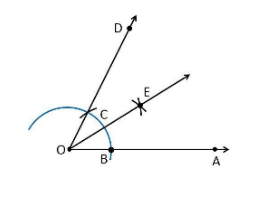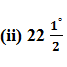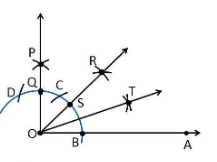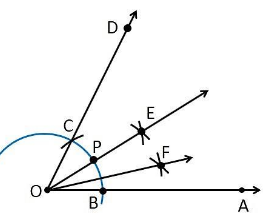Guru

# Construct the angles of the following measurements? Q.3

• 0

Please give me the best way for solving the problem of class 9th ncert math of Constructions chapter of math of class 9th of exercise 11.1of question no 3  what is the tricky way for solving this question Construct the angles of the following measurements?

Share

1. (i) 30°

Construction Procedure:

1. Draw a ray OA

2. Take O as a centre with any radius, draw an arc BC which cuts OA at B.

3. With B and C as centres, draw two arcs which intersect each other at the point E and the perpendicular bisector is drawn.

4. Thus, ∠EOA is the required angle making 30° with OA.Construction Procedure:

1. Draw an angle ∠POA = 90°

2. Take O as a centre with any radius, draw an arc BC which cuts OA at B and OP at Q

3. Now, draw the bisector from the point B and Q where it intersects at the point R such that it makes an angle ∠ROA = 45°.

4. Again, ∠ROA is bisected such that ∠TOA is formed which makes an angle of 22.5° with OA(iii) 15°

Construction Procedure:

1. An angle ∠DOA = 60° is drawn.

2. Take O as centre with any radius, draw an arc BC which cuts OA at B and OD at C

3. Now, draw the bisector from the point B and C where it intersects at the point E such that it makes an angle ∠EOA = 30°.

4. Again, ∠EOA is bisected such that ∠FOA is formed which makes an angle of 15° with OA.

5. Thus, ∠FOA is the required angle making 15° with OA.• 0